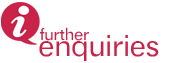﻿﻿﻿﻿﻿﻿﻿ Research Programmes | INSTITUTE FOR MATHEMATICAL RESEARCH
» LABORATORIES » Laboratory of Cryptography, Analysis and Structure » Research Programmes

# Research Programmes

1. Cryptography and Computational Number Theory

Cryptography together with Computational Number Theory is a fast developing mathematical field due to its widespread application in information security. The research encompasses mathematical ideas and subjects namely number theory, geometric algebra, chaos theory and other related fields. Amongst the widespread research areas are to find cryptographic efficient algorithms, primality testing, factoring large integers, lattice-based cryptography, sieve methods, elliptic curve cryptography discrete log problems and other related fields. Eventual aims are the development, improvement and implementation of cryptographic protocols that can become standards for communications security.

Keywords : cryptology, cryptography, cryptanalysis

2. Analytical Structural Modelling and Topological Networking

Analytical Structural Modeling and Topological Networking aims to identify and formulate the real network problems and determine the suitable method for solving. Solving the problems involve the use of proper analytical technique and studying the mathematical properties. It is considered an interdisciplinary program and focus on the upfront of future analysis and topological network

Keywords : analytical approached modeling, topological networking method

Updated:: 06/06/2017 [noorizzati]

MEDIA SHARING